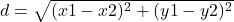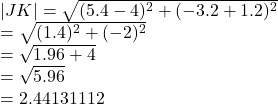## what is the distance between J(5.4,-3.2) and K(4,-1.2)

Question

what is the distance between J(5.4,-3.2) and K(4,-1.2)

in progress 0
6 months 2021-08-17T07:18:24+00:00 1 Answers 6 views 0

## 2.44 units

Step-by-step explanation:

The distance between two points can be found by using the formulawhere

(x1 , y1) and (x2 , y2) are the points

From the question the points are

J(5.4,-3.2) and K(4,-1.2)

The distance between them isWe have the final answer as

### 2.44 units

Hope this helps you